[TOC]

# 5.1 - More Functions on Lists

already known methods:

```xs.head
xs.tail
```

sublist and ele access:

• `xs.length`
• `xs.last`
• `xs.init`: all elementh except last element
• `xs.take(n)`: sublist of first n elements
• `xs.drop(n)`: the rest of list after taking first n elements
• `xs(n)`: = `xs.apply(n)` , element at index n

More methods:

• concatenation: `xs ++ ys` (`:::` is legacy usage)
• `xs.reverse`
• `xs.updated(n,x)`: return a same list, except xs(n)=x (Note: Lists are immutable, so cannot modify)
• `xs.indexOf(x)`: index or -1
• `xs.contains(x)`: same as `xs.indexOf(x)>=0`

complexity: head, tail: simple to implement ⇒ complexity of `last`?

```def last[T](xs: List[T]): T = xs match{
case List() => throw new Error("last of Nil")
case List(x) => x
case y::ys => last(ys)
}
```

⇒ complexity of last = O(n)

implement `init`:

```def init[T](xs: List[T]): T = xs match{
case List() => throw new Error("init of Nil")
case List(x) => List()
case y::ys => y::init(ys)
}
```

⇒ complexity = O(length of xs)

implement concatenation `:::`/`++` (NB: `:::` is right-associative, `xs:::ys` = `ys. :::(xs)`. )

```def concat[T](xs: List[T], ys: List[T]) = xs match{
case List() => ys
case z::zs => x:concat(xs, ys)
}
```

⇒ complexity of concat = O(length of xs)

implement of reverse

```def reverse[T](xs: List[T]) = xs match{
case List() => List()
case y::ys => reverse(ys) ++ y
}
```

⇒ complexity of reverse: every call contains a concat, thus complexity=O(n2)

exercice: remove nth element:

```def removeAt[T](n:Int, xs: List[T]): List[T] =
(xs take n ) ++ (xs drop n+1)
```

# 5.2 - Pairs and Tuples

example: sort list faster than insertion sort → merge sort.

sort 2 sublist, merge them ⇒ list is sorted

```def msort(xs: List[Int]): List[Int] = {
val n = xs.length/2
if(n==0) xs
else{
def merge(xs: List[Int], ys: List[Int]) = ... // see below
val (fst, snd) = x splitAt n // splitAt returns 2 sublists
merge(msort(fst), msort(snd))
}
}
```

merge:

```def merge(xs: List[Int], ys: List[Int]): List[Int] = xs match {
case Nil => ys
case x::zs => match ys{
case Nil => xs
case y::ws => {
if(x<y) x::merge(zs, ys)
else y::merge(xs, ws)
}
}
}
```

### Pair/Tuple

written as `(x, y)` in scala. pair can be used as patterns : similar for tuples.

```val pair = ("a", 2)
val (label, value) = pair
```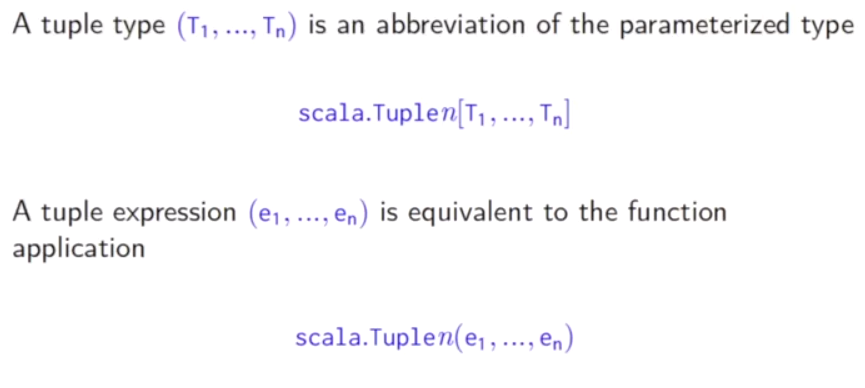tuple implementation: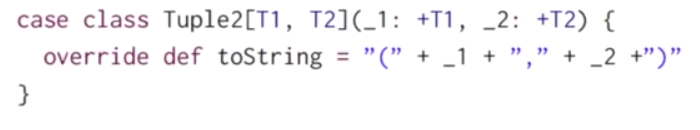⇒ can use `_1` `_2` to access elements

exercice: rewrite the merge function using a pattern matching over pairs:

```def merge(xs: List[Int], ys: List[Int]): List[Int] =
(xs, ys) match {
case (Nil, ys) => ys
case (xs, Nil) => xs
case (x:zs, y:ws) =>
if(x<y) x::merge(zs, ys)
else y::merge(xs, ws)
}
```

# 5.3 - Implicit Parameters

pb: how to apply msort to list of other element types.

using type parameters ? `msort[T]`⇒ the compare operator is not always defined !

pass the lt function as a parameter:

`def msort[T](xs: List[T])(lt: (T,T)=>Boolean) = ...`

another option: `scala.math.Ordering[T]`

```impor math.Ordering
def msort[T](xs: List[T])(ord: Ordering) = ...// use ord.lt(x,y)
msort(nums)(Ordering.Int)
```

pb: pass each time the function parameter is cumbersome... ⇒ use implicite parameters

```def msort[T](xs: List[T])(implicite ord: Ordering) = ...// use ord.lt(x,y)
```

⇒ the function calls can ignore the implicite parameter, the compiler will figure it out.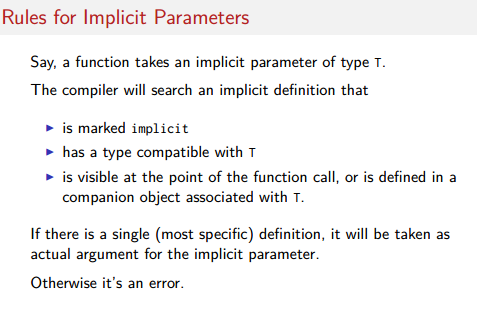# 5.4 - Higher-Order List Functions

functions over list have similar pattern:

• transform each element
• retrive elements that satisfy some cretirion
• combing elements using an operator

### map

apply an operation to every elements.

```abstract class List[T]{
def map[U](f: T=>U): List[U] = this match {
case Nil => this
case x:xs => f(x)::xs.map(f)
}
}
```

### filtering

```def filter(p: T=>Boolean): List[T] = this match {
case Nil => this
case x:xs => f(p(x)) x::xs.filter(p) else xs.filter(p)
}
```

other methods that extracts sublist: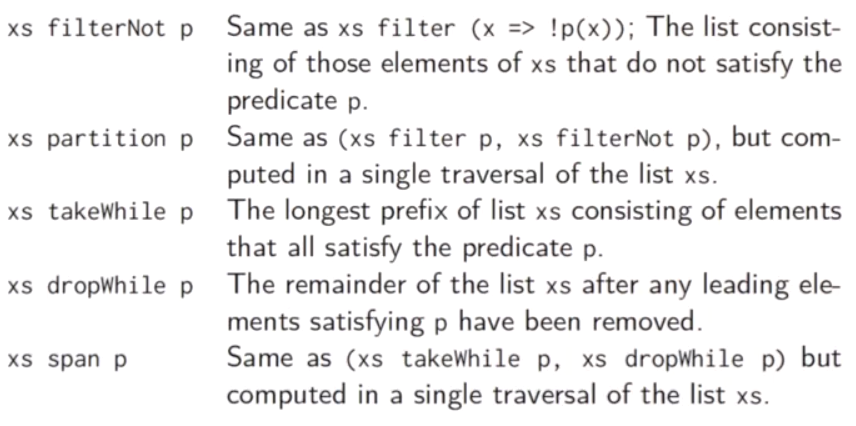exercice: implement a function `pack`: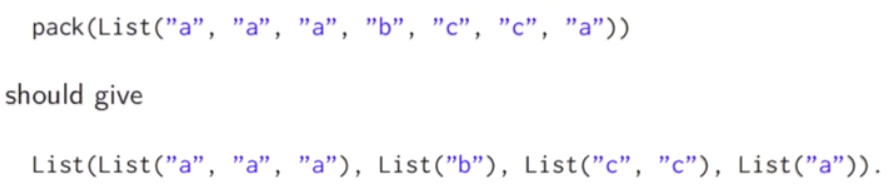```def pack[T](xs: List[T]): List[List[T]] = xs match {
case Nil => Nil
case x::ys => {
val (head, tail) = xs span (c => c==x)
head :: pack(tail)
}
}
```

exercice2: implement a function `encode`: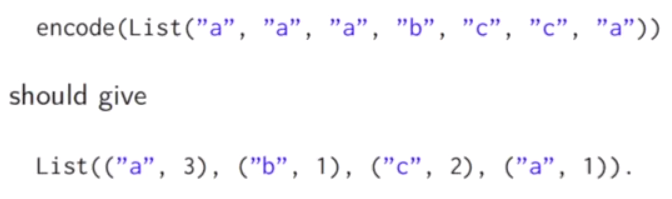```def encode[T](xs: List[T]): List[(T, Int)] = xs match {
case Nil => Nil
case x::ys => {
val (head, tail) = xs span (c => c==x)
(x, head.length) :: pack(tail)
}
}
```

another version: use the pack:

```def encode[T](xs: List[T]): List[(T, Int)] =
pack(xs) map (l => (l.head, l.length))
```

# 5.5 - Reduction of Lists

fold/reduce: combine elements using an operator.

### reduceLeft

(can apply only to non-empty lists) inserts a binary operator between adj elements: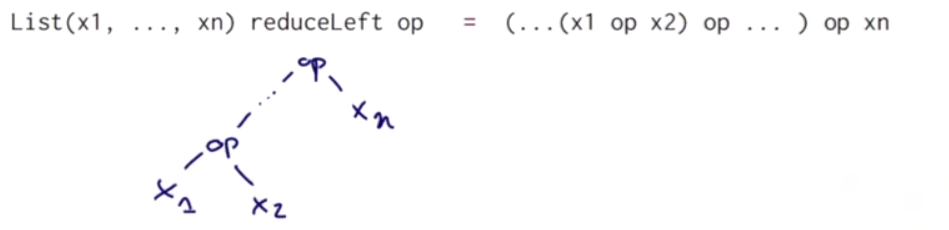ex.

```def sum(xs: List[Int]) = (0::xs) reduceLeft ( (x,y)=> x+y)
def prod(xs: List[Int]) = (1::xs) reduceLeft ( (x,y)=> x*y)
```

write shorter function values using underscore `_`: every `_` represents a new parameter

```def sum(xs: List[Int]) = (0::xs) reduceLeft ( _+_ )
def prod(xs: List[Int]) = (1::xs) reduceLeft ( _*_ )
```

### foldLeft

foldLeft is like reduceLeft, but can apply on Nil, and takes an accumulator `z` => returns z when calling on Nil.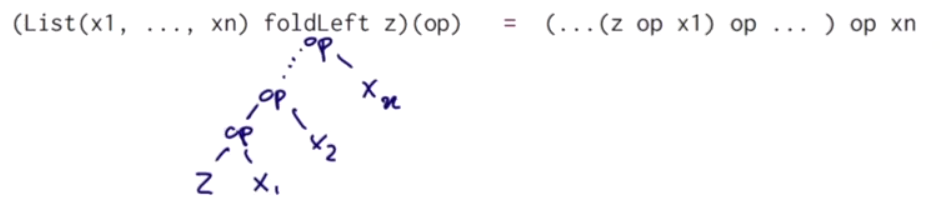```def sum(xs: List[Int]) = (xs foldleft 0) ( _+_ )
def prod(xs: List[Int]) = (xs foldleft 1) ( _*_ )
```### foldRight/reduceRight

dual functions to `foldLeft` and `reduceLeft`, but produce a tree leaned to right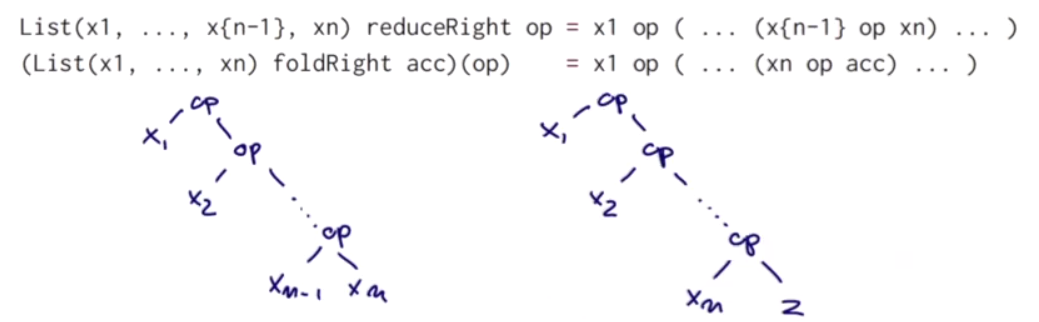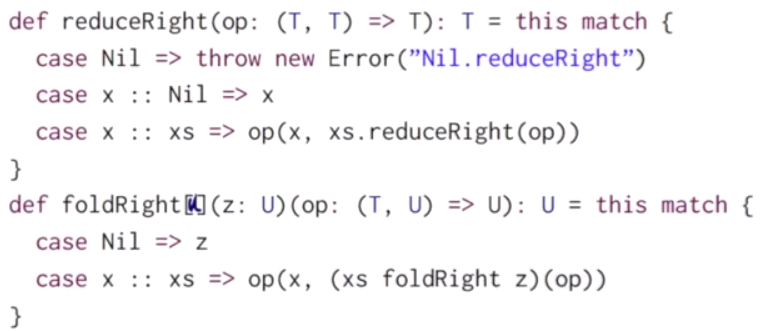if the operation is associative and communitive, foldLeft and foldRight should give same results. Other times need to think.

ex. concat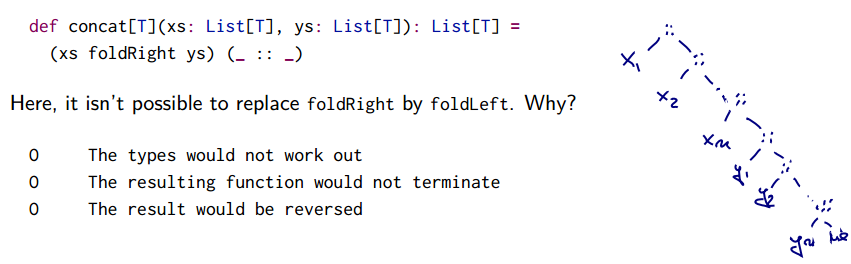if apply foldLeft ⇒ type error, because the `::` operator will be applied to 2 elements of type T.

# 5.6 - Reasoning About Concat

proof of programs

### structural induction

pb: prove some properties of concat:ex. prove `(xs ++ ys) ++ zs = xs ++ (ys ++ zs)`: induction on `xs`

```def concat[T](xs: List[T], ys: List[T]) = xs match{
case Nil => ys
case z::zs => x:concat(xs, ys)
}
```
• base case: `xs=Nil`

`(Nil ++ ys ) ++ zs = Nil ++ (ys ++ zs)`

• induction step: `x::xs`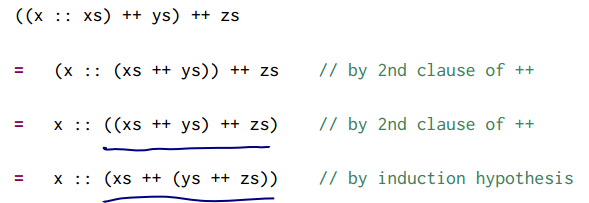## 5.7 - A Larger Equational Proof on Lists

pb: want to prove that `xs.reverse.revese == xs`

• base case: `Nil.reverse.revers = Nil`
• induction step

pb: cannot advance ⇒ generalize the argument.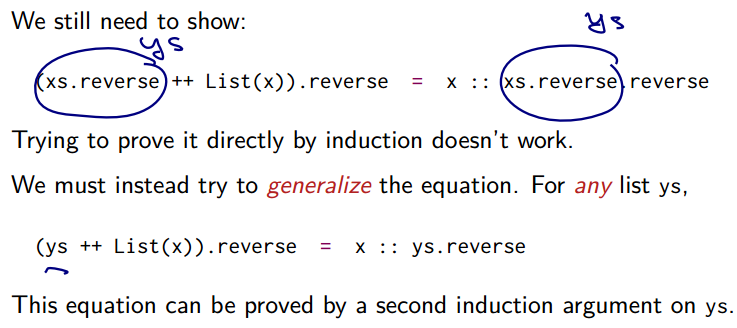#### Part 6 of series «Functional Programming Principles in Scala»：

comments powered by Disqus